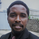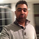30093 views
30093
This strategy combines the classic RSI strategy to sell when the RSI increases over 70 (or to buy when it falls below 30), with the classic Stochastic Slow strategy to sell when the Stochastic oscillator exceeds the value of 80 (and to buy when this value is below 20).

This simple strategy only triggers when both the RSI and the Stochastic are together in a overbought or oversold condition. The one hour chart of the S&P 500 worked quite well recently with this double strategy.

By the way this strategy should not be confused with the 'Stochastic RSI', which measures the RSI only.

All trading involves high risk; past performance is not necessarily indicative of future results.
//@version=2
strategy("Stochastic + RSI, Double Strategy (by ChartArt)", shorttitle="CA_-_RSI_Stoch_Strat", overlay=true)

// ChartArt's Stochastic Slow + Relative Strength Index, Double Strategy
//
// Version 1.0
// Idea by ChartArt on October 23, 2015.
//
// This strategy combines the classic RSI
// strategy to sell when the RSI increases
// over 70 (or to buy when it falls below 30),
// with the classic Stochastic Slow strategy
// to sell when the Stochastic oscillator
// exceeds the value of 80 (and to buy when
// this value is below 20).
//
// This simple strategy only triggers when
// both the RSI and the Stochastic are together
// in overbought or oversold conditions.
//
// List of my work:

///////////// Stochastic Slow
Stochlength = input(14, minval=1, title="lookback length of Stochastic")
StochOverBought = input(80, title="Stochastic overbought condition")
StochOverSold = input(20, title="Stochastic oversold condition")
smoothK = input(3, title="smoothing of Stochastic %K ")
smoothD = input(3, title="moving average of Stochastic %K")
k = sma(stoch(close, high, low, Stochlength), smoothK)
d = sma(k, smoothD)

///////////// RSI
RSIlength = input( 14, minval=1 , title="lookback length of RSI")
RSIOverBought = input( 70  , title="RSI overbought condition")
RSIOverSold = input( 30  , title="RSI oversold condition")
RSIprice = close
vrsi = rsi(RSIprice, RSIlength)

///////////// Double strategy: RSI strategy + Stochastic strategy

if (not na(k) and not na(d))
if (crossover(k,d) and k < StochOverSold)
if (not na(vrsi)) and (crossover(vrsi, RSIOverSold))
strategy.entry("LONG", strategy.long, comment="StochLE + RsiLE")

if (crossunder(k,d) and k > StochOverBought)
if (crossunder(vrsi, RSIOverBought))
strategy.entry("SHORT", strategy.short, comment="StochSE + RsiSE")

//plot(strategy.equity, title="equity", color=red, linewidth=2, style=areabr)Hello,
I'm new to trading and MT4. Please can some one be kind enough to explain how I can compile this script on MQL.
Thank you